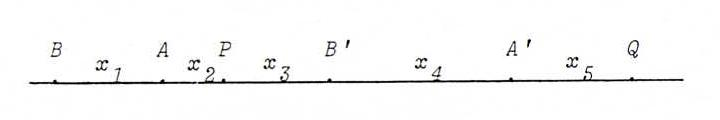### PROJECTIVE GEOMETRY COURSE

§ 14: Involutions

Definition : We call a non-trivial projectivity φ from a line l onto itself (or from a pencil L onto itself) involution if φ2 is identity.

Proposition : If a projectivity φ from l onto itself (or from L onto itself) interchanges two points (lines), then φ is an involution.

Proof : Suppose that φ interchanges the points A and B, and let X be a third point on l. Then (A, B; X, φ(X)) = (φ(A), φ(B); φ(X), φ2(X)) = (B, A; φ(X), φ2(X)).
We also have (A, B; X, φ(X)) = (B, A; φ(X), X). So according to O38 we must have φ2(X) = X.

Remark : We call {X, φ(X)} a pair of the involution.

Proposition : If an involution φ has a fixed point P (invariant line p), then φ has another fixed point RP (invariant line rp), and the pairs of φ separate {P,R} harmonically.

Proof : Let {X,φ(X)} be a pair of φ with X ≠ φ(X). Let R be the fourth harmonic with P and {X,φ(X)}. We have to prove that φ(R) = R. (Then it follows that each X yields the same R, because a non-trivial projectivity can have at most two fixed points.)
Indeed: (P, R; X, φ(X)) = -1, so (P, R; X, φ(X)) = (R, P; X, φ(X)). Also (P, R; X, φ(X)) = (φ(P), φ(R); φ(X), φ2(X)) = (P, φ(R); φ(X), X) = (φ(R), P; X, φ(X)).
According to O38, we find φ(R) = R.

Remark : So we can construct with each point on l (each line through L) the image point (the image line) if the fixed points (invariant lines) are given (see O49).

Remark : The proposition implies that there doesn't exist any parabolic involution.
The involution with pairs {A,A'} and {B,B'} is elliptic if {A,A'} and {B,B'} separate. Indeed, suppose that this involution is hyperbolic with fixed points P and Q.Because of the harmonic positions with a hyperbolic involution we would have:
x2/(x2+x3+x4+x5) = (x3+x4)/x5 en (x1+x2)/(x1+x2+x3+x4+x5) = x3/(x4+x5).
A contradiction follows, because the first number is smaller than the third (check this) and the second is greater than the fourth.

O55 Determine the matrix of the involution with pairs {(4,-5),(1,2)} and {(5,-4),(2,1)}.

O56 When is ρ((a,b),(c,d)) the matrix of an involution? When is it hyperbolic, when elliptic?

O57 Suppose r(X1, ...) _ s(X2, ...) with Pappus line p, and s(X2, ...) = r(X3, ...) with center P.
Prove that the product r(X1, ...) _ r(X3, ...) is an involution if and only if P lies on p.

O58 Suppose we have a pencil of points l on which the pair {P,Q} separates both the pair {A,A'} and the pair {B,B'} harmonically.
Prove that {A',B'} is a pair of the involution with pairs {P,Q} and {A,B}.